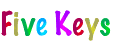Multiplying Fractions with Fraction Circles

Unlike the rules for adding and subtracting fractions, the rule for multiplying fractions is what the numbers suggest; just multiply across: numerator x numerator and denominator x denominator. It is the one rule that students tend to remember. It is also the one that leads them to just add across or subtract across when adding or subtracting fractions, so they should multiply some fractions with Fraction Circles to see the difference in thinking.

When used with fractions, the multiplication sign takes on the meaning of the word “of. For example, 1/2 x 2/3 means 1/2 of 2/3, which is 1/3. This is due to how fractional parts are expressed in daily living. When we say “half of ten, the math being referred to is 1/2 x 10 = 1/2 x 10/1 = 1 x 10/2 x 1 = 10/2 = 5. The following lesson capitalizes on this understanding of the times sign when used with fractions.

Dividing Fractons with Fraction Circles

The rule for dividing fractions is one of the weirdest of all rules in math: “To divide fractions, invert the divisor and multiply. It’s been around for so many decades that it is passed on as if it were wisdom, not math. Because of that, why it works is rarely explained, but because it is math, it should be. The proof for it is short and elegant and is included with this key. It is attributed to Mr. Novak—a math teacher in a TV series that was popular in the 60s—who explained it in one of the programs. Sadly, his explanation was for a class of high school seniors. It should have been for students still in elementary school.

The lesson for dividing fractions with Fraction Circles sequences through three sets of six problems each. Each set yields a rule that works for certain fractions:

• The first set yields the rule to divide the second denominator by the first one. For example, for 1/4 ÷ 1/16 (which equals 4), 16 ÷ 4 = 4, but the rule only works if both numerators are 1 and the answer is a whole number.

• The second set yields the rule to divide the second denominator by the first one and multiply the quotient by the first numerator. For example, for 4/5 ÷ 1/10 (which equals 8), 10 ÷ 5 = 2 and 2 x 4 = 8, but the rule only works if the second numerator is 1 and the answer is a whole number.

• The third set yields the rule to divide the second denominator by the first one and multiply the quotient by the first numerator and divide the product by the second numerator. For example, for 4/5 ÷ 2/15 (which equals 6), 15 ÷ 5 = 3, 3 x 4 = 12, and 12 ÷ 2 = 6, but the rule only works if the answer is a whole number.

Since none of the rules work for all fractions, present the “invert and multiply rule and have students verify that it works by using it to rework problems 1-18. Conclude with Mr. Novak’s proof of the rule.

Remembering Forgotten Rules

Here are the steps again that lead to figuring out the ASMD rules for fractions:

• Students add, subtract, multiply, and divide fractions with Fraction Circles to obtain answers that they know are correct because they can see that they are.

Students then examine the numbers in the problems they solved and the numbers in the answers they got to find a rule to get the answers they got using just the numbers in the problems.

Here are the steps reworded for remembering a rule if forgotten:

• Have students pretend that they are not sure of a rule (whether they are or not) and that they don’t have access to the Fraction Circles (because they normally wouldn’t).

CONTINUED: 1 | 2 | 3 | 4 | 5 | 6 | 7 | < Back